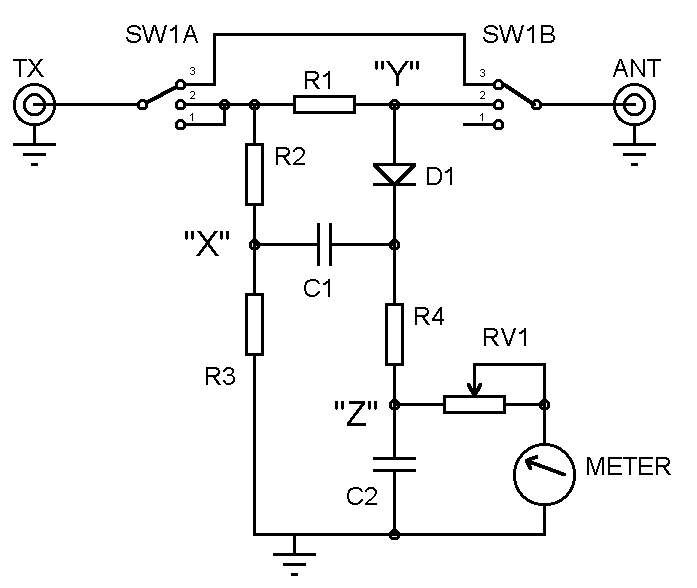The Resistive SWR Bridge
 The resistive SWR bridge is highly recommended for both beginners and experienced constructors. It is simple, inexpensive to build, can be very sensitive and is therefore highly suited for QRP operation. When the bridge is set to position 2 to enable tuning of the Antenna System Matching Unit (ASMU), the transmitter P.A. stage will always see a lower SWR than is present at the load. Indeed, if it’s a dead short, or open circuit the transmitter will only see a 3:1 SWR. Other complex antenna impedance’s may present a higher SWR to the antenna socket, but the rig will always see one that is lower and therefore provide some protection to your P.A. transistor. Because the design relies on using a resistive bridge, the transmitter output should be limited by how much power the resistors can safely dissipate. Please note that inductive (i.e. wire wound) resistors should not be used. Ian Keyser G3ROO explains how it works....Considering the following statements will help with the following explanation:- In the interest of simplicity we will assume that the meter draws no current and there is no phase difference between points X and Y. When RF is applied to the input of the ‘bridge’ the potential at point X will be at half the total RF potential applied to the bridge, and this will remain so at all times. D1, C1, R4 and C2 form a peak voltage detector. Reading the potential at point Y in relation to point X. We will consider three possible scenarios, In switch position 1 no load (i.e. antenna) is applied and so the bridge is now reading the peak RF input voltage via R1 [50 Ohms], this will have no noticeable effect on the reading. This position can be used to adjust VR1 for Full Scale Deflection. In switch position 2 and with a load of high impedance the RF potential at point Y is very nearly the same as the input RF voltage. In this instance the peak DC voltage at point Z will be almost half the peak RF voltage applied (RF volts input - RF Volts/2 [point X]). Alternatively, if the load impedance is now made very low (say 1 Ohm), RF volts at point Y is almost zero so the peak detector will again read about half the RF input voltage - (RF volts input/2 [point X] - point Y (zero volts [almost]). Between the above two extremes, if the load impedance is 50 Ohms, the voltage at point Y will now be half input RF voltage. As now point X and Y are at half RF input voltage the potential difference between them is zero. This is the point of 1:1 SWR, and as we deviate from this ideal, the voltage detected will rise until the two other scenarios are reached. Having tuned the ASMU (with the switch in position 2), to indicate 1:1 (zero volts), the switch should now be moved to position 3 to remove the bridge and it will connect the ASMU directly to the transmitter. To leave it in circuit in position 2 would divide the R.F power between the antenna and the resistors in the bridge. The aspect that catches most out is the fact that the meter is connected to ground and not to point X. The reason for this is that as far as the meter is concerned it is only interested in indicating the DC component. If it is connected to earth, VR1 has only to be reduced in value by the value of R3 to give the same reading. In practice, as VR1 is going to be in the order of kilo ohms compared to the 51 ohms of R3, this error can be ignored. Parts List: RV1 10K, R1/R2/R3 51 Ohm (2W), R4 1K (0.5W), C1/2 10nF, D1 OA91 (or similar), Meter 1mA FSD, SW1 2 pole 3 way